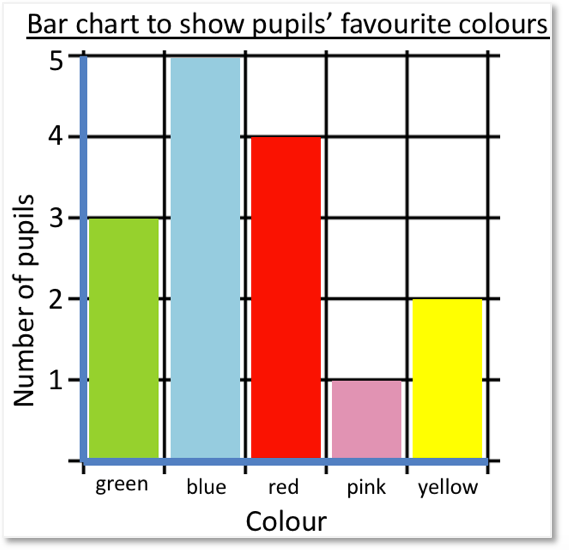How to Find the Coordinates of a Point

How to Find the Coordinates of a Point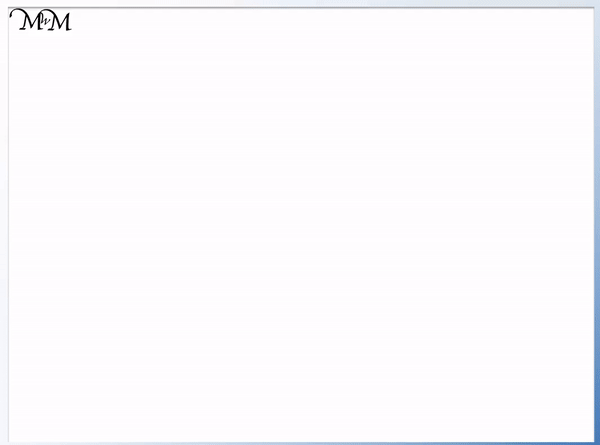• A pair of coordinates are two numbers that tell us the location of a point on a grid.
• Coordinates are two numbers written in between brackets, separated by a comma.
• The first number is called the x-coordinate and tells us how far to the right our point is.
• The second number is called the y-coordinate and tells us how far up our point is.
• To help us remember the order we can say ‘along the corridor, up the stairs’.
• This helps us remember that the first number tells us how far along (right) and the second number tells us how far up.
• We need to write down the two numbers of our coordinate pair in this order.
Coordinates are written as two numbers separated by a comma, inside brackets.

The first number tells us how far to the right right and the second number tells us how far up our point is.• Our point is marked on our grid with a cross on the grid lines.
• We write our coordinate inside a set of brackets.
• We will write down how far to the right and how far up our point is from zero.
• We first read ‘along the corridor’ to count how far to the right our point is. This is the x-coordinate.
• The x-coordinate is 4 and we write this first.
• Now we read ‘up the stairs’ to count how far up our point is. This is the y-coordinate.
• The y-coordinate is 9.
• The coordinate of our point is (4,9).How to Find the Coordinates of a Point

A pair of coordinates are two numbers that tell us the exact position of a point that is plotted on a grid.

The most common grid that we see is called the Cartesian plane. The Cartesian plane that we will consider is a

grid that contains an x-axis and a y-axis.

The x-axis is the horizontal line and the y-axis is the vertical line. They are at right angles to each other.

These two

are labelled on the image below. We can see that there are numbers equally spaced along each axis, almost like a number line. The numbers on each axis are written in line with the grid lines.In the bottom left corner of this grid we can see where the x-axis and y-axis meet. This place is called the origin.

The numbers in this bottom left corner on each axis are both zero.Finding the coordinates of a point means that we write down the location of the point using numbers.

To find the coordinates of any point drawn on our grid, we start at the origin and read how far to the right the point is and how far up a point is. We write these two distances in order, separated by a comma, in between a set of brackets.Above is a point on our Cartesian plane. We typically plot a coordinate point with a cross and it should be drawn directly on the grid lines.

Remember that the coordinates describe how far to the right a point is and how far up it is from the origin.

We must give these coordinate numbers in this order.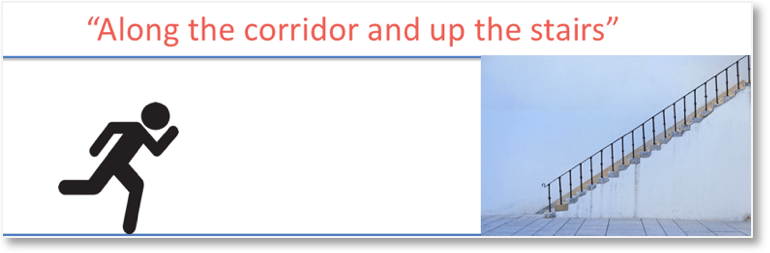When teaching reading coordinates, we can use the phrase

“along the corridor and up the stairs”

to remind us that we first describe how far to the right the point is from zero, and then how far up it is from zero.

The ‘along the corridor’ represents us going along (to the right) and the ‘up the stairs’ represents going up.

This phrase is commonly used in schools when teaching coordinates because it helps to avoid the most common mistake of writing the two numbers in the wrong order.We will write the coordinates in brackets, which are shown in the image above.

Remember that the coordinates are formed from two numbers, separated by a comma.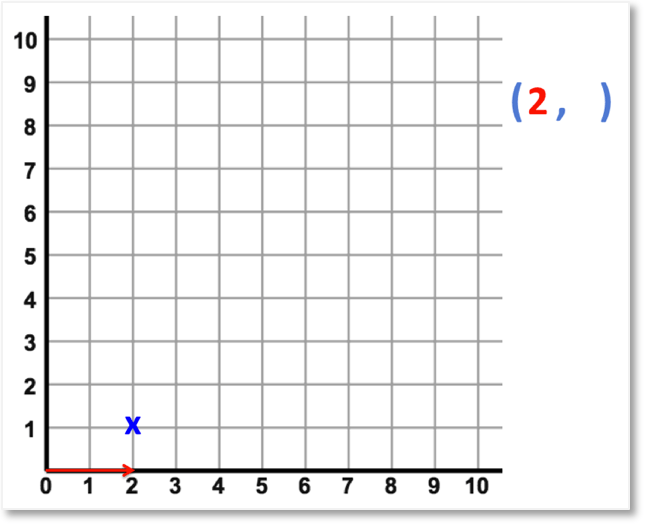We begin by going “along the corridor”. So, we read how far to the right we are from the origin first.

The point is 2 places along to the right from zero. So, we write 2 in the brackets as our first number in our coordinate pair.

Because we read the ‘2’ from our x-axis, we call this number our x-coordinate.

The x-coordinate is the first number in our pair of coordinates.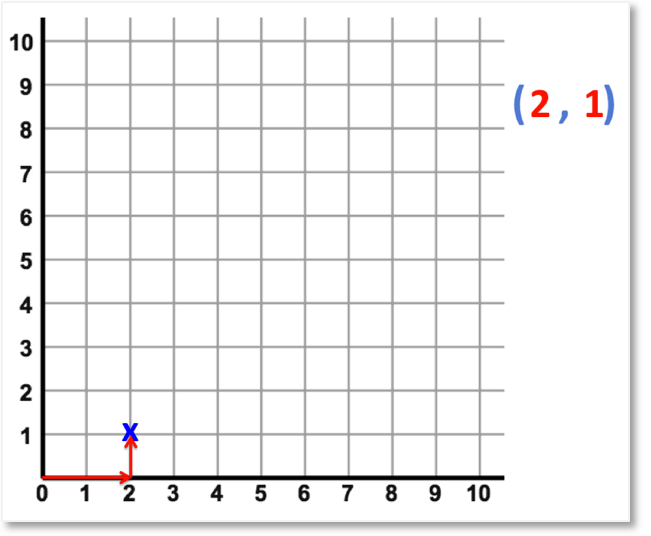Having moved 2 to the right, we now go “up the stairs” to get to our point. So, now we read how many places we move up to get to our point.

From the x-axis, we have moved 1 place up the grid.

We write ‘1’ as the second number in the brackets, after the comma.

This second number after the comma is known as the y-coordinate.

Therefore our coordinate pair is made up of two numbers: the x-coordinate, followed by the y-coordinate.

The order of coordinate numbers matters.

We always write the x-coordinate first and then y-coordinate after.

In our example, we have moved 2 places right and 1 place up. We have shown this with the coordinates (2,1).

We write these numbers in brackets and separate them with a comma to form a coordinate pair.

This helps mathematicians because we know that when we see two numbers, separated by a comma, inside brackets that we probably are talking about a coordinate.We must make sure that we write the numbers for our coordinates in the correct order.

As you can see above, if we wrote (1,2) instead of (2,1), it would mean that we would go right 1 place and up 2 places, instead of right 2 places and up 1 place.

Remember that when teaching coordinates, the most common mistake is writing the numbers in the wrong order. It is worth showing a comparison of two coordinates with the numbers reversed such as in the example above, showing that we are describing two separate locations.

Here’s another example of reading Cartesian coordinates:To find out what the coordinates are, we can use “along the corridor and up the stairs”.Remember that we want two numbers to describe the location.

The x-coordinate tells us how far to the right and the y-coordinate tells us how far up.

Remember that we use ‘along the corridor and up the stairs’ to help us remember the order to write these numbers.

First, we read in the horizontal direction. So, we see how many places to the right it is.

We can see that it is 3 places right. We write 3 as the first number in our brackets.Next, we read in the vertical direction. So, we count how many places up from zero it is.

We can see that it is 8 places up. We write the number 8 in the brackets, after the comma.

We have moved 3 places right and 8 places up from the origin to get to our point.

We have shown this by writing the the coordinates (3,8).Now try our lesson on Drawing and Interpreting Bar Charts where we learn how to draw bar charts to represent a set of data.